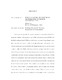## ERROR ANALYSIS OF NUMERICAL METHODS FOR NONLINEAR GEOMETRIC PDEs##### Files
Li_umd_0117E_19829.pdf(3.48 MB)
(RESTRICTED ACCESS)
This dissertation presents the numerical treatment of two classes of nonlinear geometric problems: fully nonlinear elliptic PDEs and nonlinear nonlocal PDEs. For the fully nonlinear elliptic PDEs, we study three problems: Monge-Amp\{e}re equations, computation of convex envelopes and optimal transport with quadratic cost. We develop two-scale methods for both the Monge-Amp\{e}re equation and the convex envelope problem with Dirichlet boundary conditions, and prove rates of convergence in the $L^{\infty}$ norm for them. Our technique hinges on the discrete comparison principle, construction of barrier functions and geometric properties of the problems. We also derive error estimates for numerical schemes of the optimal transport problem with quadratic cost, which can be written as a so-called second boundary value problem for the Monge-Amp\`{e}re equation. This contains a new weighted $L^2$ error estimate for the fully discrete linear programming method based on quantitative stability estimates for optimal plans. For the nonlinear nonlocal PDEs, we focus on the computation and numerical analysis of nonlocal minimal graphs of order $s \in (0,1/2)$ in a bounded domain. This can be reinterpreted as a Dirichlet problem for a nonlocal, nonlinear, degenerate operator of order $s + 1/2$, whose numerical treatment is in its infancy. We propose a finite element discretization and prove its convergence together with error estimates for two different notions of error. Several interesting numerical experiments are also presented and discussed, which might shed some light on theoretical questions about this emerging research topic.